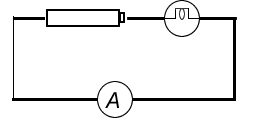#### Measuring Current Laboratory

Purposes:

1. To observe and measure the flow of current in a series circuit

2. To learn how to properly use an ammeter to measure current in a circuit

Materials & Apparatus:

• batteries and battery holders

• ammeter

• connecting wires with alligator clips

• light bulbs and base holders

• switch (optional)

Procedure:

a) single bulb

1. Set up the following circuit diagram

2. Draw the equivalent circuit diagram using proper symbols

3. Measure the current in the circuit

4. Record the value of currentCurrent = __________________

b) two cells in series

Repeat steps 1 to 4 aboveCurrent = _______

Questions:

1. What are the general rules for connecting ammeters in a circuit.

2. What can you conclude about the current in a series circuit?

3. Questions 1-7 p327 (your textbook)